Author: Oscar Cronquist Article last updated on October 31, 2018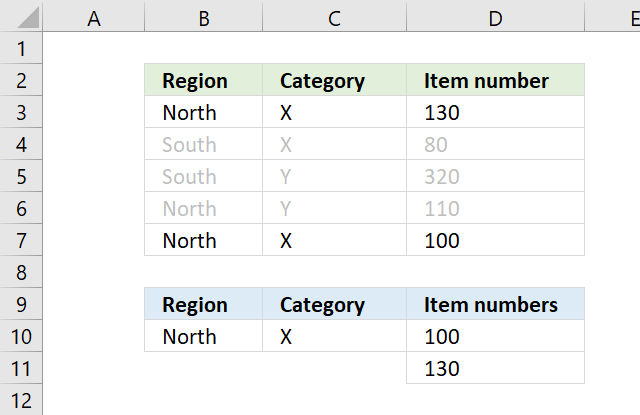The array formula in D10 extracts numbers sorted from small to large from column D if Region is equal to North AND Category is X on the same row.

=SMALL(IF((\$B\$10=\$B\$3:\$B\$7)*(\$C\$10=\$C\$3:\$C\$7), \$D\$3:\$D\$7, ""), ROWS(\$A\$1:A1))

To enter an array formula, type the formula in a cell then press and hold CTRL + SHIFT simultaneously, now press Enter once. Release all keys.

The formula bar now shows the formula with a beginning and ending curly bracket telling you that you entered the formula successfully. Don't enter the curly brackets yourself.

There are two logical expressions in the formula returning boolean values TRUE or FALSE.

(\$B\$10=\$B\$3:\$B\$7)*(\$C\$10=\$C\$3:\$C\$7)

The asterisk between the logical expressions multiply the boolean arrays, this will help us extract numbers where both conditions match on the same row.

{TRUE;FALSE;FALSE;TRUE;TRUE}) * ({TRUE;TRUE;FALSE;FALSE;TRUE}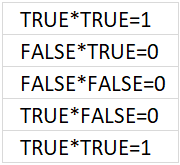and returns {1;0;0;0;1}.

The IF function then returns the corresponding values from column D if TRUE and a blank "" if FALSE.

IF((\$B\$10=\$B\$3:\$B\$7)*(\$C\$10=\$C\$3:\$C\$7), \$D\$3:\$D\$7, "")

becomes

IF({1;0;0;0;1}, {130;80;320;110;100}, "")

and returns {130;"";"";"";100}.

The SMALL function returns the k-tk smallest value the array determined by ROWS(\$A\$1:A1).

The cell reference in ROWS(\$A\$1:A1) expands when you copy the cell and paste it to cells below.

The smallest value is shown in D10, ROWS(\$A\$1:A1) returns 1. The second smallest value is shown in D11, ROWS(\$A\$1:A2) returns 2.

The ROWS function has a great advantage, it won't break the formula if you insert or delete rows above the array formula.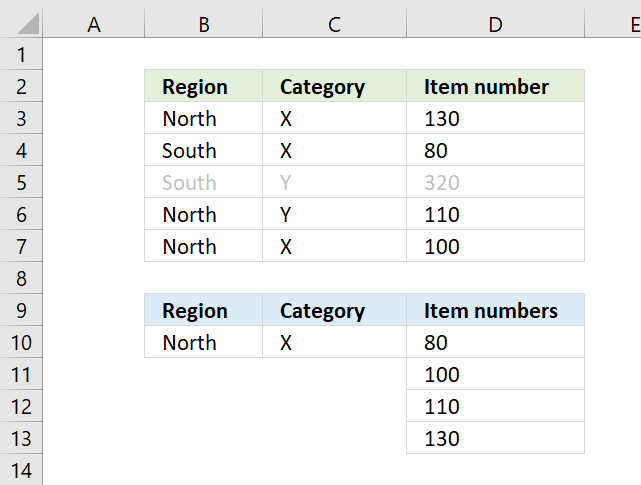The array formula in D10 extracts numbers sorted from small to large from column D if Region is equal to North OR Category is X.

=SMALL(IF((\$B\$10=\$B\$3:\$B\$7)+(\$C\$10=\$C\$3:\$C\$7), \$D\$3:\$D\$7, ""), ROWS(\$A\$1:A1))

The only difference between this formula and the formula above is the plus sign instead of the asterisk.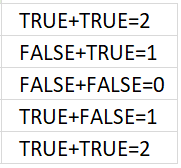If you add the logical expressions you get what the image above shows. All numbers except 0 (zero) equal TRUE and 0 (zero) is FALSE.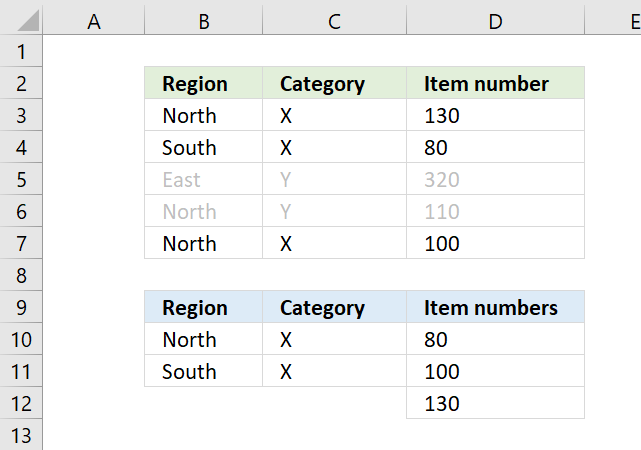The formula above in D10 extracts numbers sorted from small to large if both Region and Category match on the same row in both tables.

This formula is similar to the first formula, however, the COUNTIFS function allows you to easily use multiple criteria without building huge formulas.

=SMALL(IF(COUNTIFS(\$B\$10:\$B\$11,\$B\$3:\$B\$7, \$C\$10:\$C\$11,\$C\$3:\$C\$7), \$D\$3:\$D\$7, ""), ROWS(\$A\$1:A1))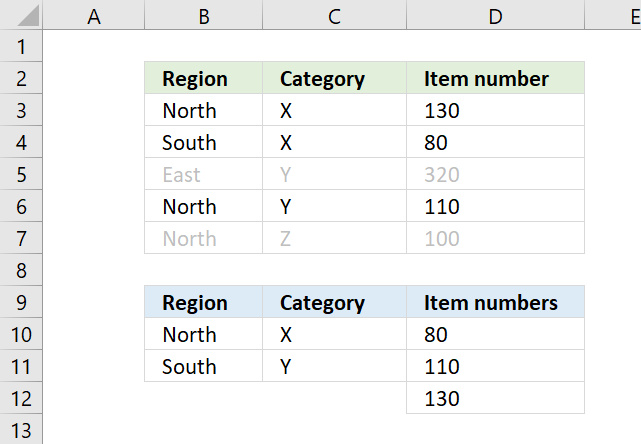The formula above in D10 extracts numbers sorted from small to large if both Region and Category match any of the criteria.

Example, Z on row 7 is not a match to any of the Category values in the lower table, both values must match.

=SMALL(IF(COUNTIF(\$B\$10:\$B\$11, \$B\$3:\$B\$7)*COUNTIF(\$C\$10:\$C\$11, \$C\$3:\$C\$7), \$D\$3:\$D\$7, ""), ROWS(\$A\$1:A1))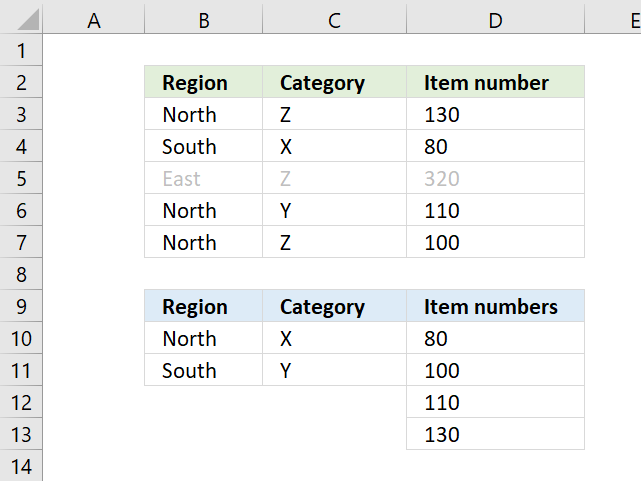The formula above in D10 extracts numbers sorted from small to large if any of the Region or Category values match.

=SMALL(IF(COUNTIF(\$B\$10:\$B\$11, \$B\$3:\$B\$7)+ COUNTIF(\$C\$10:\$C\$11, \$C\$3:\$C\$7), \$D\$3:\$D\$7, ""), ROWS(\$A\$1:A1))